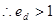# Broadly discuss the measurements of price elasticity of demand.Posted by

Last updated on June 13th, 2020 at 08:31 pm

## Measurement of Price Elasticity of Demand:

There are 5 different measurements of Price Elasticity of Demand. They are as follows:

1. Perfectly Inelastic Demand (Elasticity = 0)
2. Perfectly Elastic Demand (Elasticity = Infinity)
3. Unit Elasticity of Demand (Elasticity = 1)
4. Relatively Inelastic Demand (Elasticity < 1)
5. Relatively Elastic Demand (Elasticity > 1)

These measurements has been discussed here. In the following discussion 5 symbols has been used which are

## 1. Perfectly Inelastic Demand (Elasticity = 0):

In that given figure, price is measured on the vertical axes and the quantity demanded on the horizontal axes. It is found that the change in price has no effect on the quantity demanded. This is known as perfectly inelastic demand. Here the demand curve is vertical.Example: Taking the price is changing from \$10 to \$12 and the quantity is remaining constant at 10 units. Then,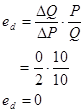## 2. Perfectly Elastic Demand (Elasticity = Infinity):

In that given figure, price is measured on the vertical axes and the quantity demanded on the horizontal axes. Here, we can find that the demand curve DD is horizontal. SO the demand is perfectly elastic.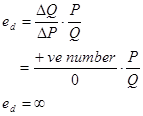Example: Taking that the price is remaining constant at \$10 and the demand is changing from 20 units to 30 units. Then,## 3. Unit Elasticity of Demand (Elasticity = 1):

In that given figure, price is measured on the vertical axes and the quantity demanded on the horizontal axes. Here, we can see that the change in demand is exactly equal to the change in price. That is, if there is 20% change in price then there will be 20% change in demand in the case of unit elasticity of demand.Example: Taking that there is a change of price from \$100 to \$120 and a change in demand from 1000 units to 800 units. Then,## 4. Relatively Inelastic Demand (Elasticity < 1):

In that given figure, price is measured on the vertical axes and the quantity demanded on the horizontal axes. In that case the change in price will be more than the change in demand. In the figure, it can be found that theis more than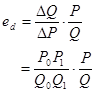Thus,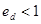This is relatively inelastic demand.

Example: Taking that the price is changing from \$100 to \$150 and demand is changing from 1000 units to 1200 units. Then,## 5. Relatively Elastic Demand (Elasticity > 1):

In that given figure, price is measured on the vertical axes and the quantity demanded on the horizontal axes. In that case the change in price will be less than the change in demand. In the figure, thatis less thanThis is relatively elastic demand.

Example: Taking that there is a change of price from \$100 to \$120 and a change in demand from 1000 units to 600 units. Then,